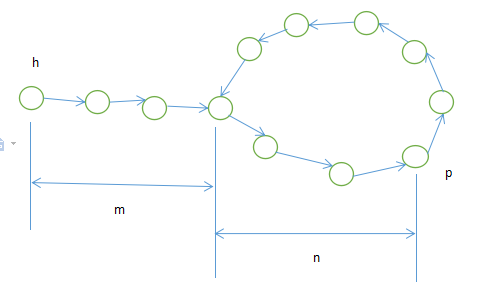# 判圈算法(Flyod、Brent's)

## Floyd cycle detection（龟兔算法）

### 环起点

#### 分析int *t = head, *h = head;
// 判圈
do{
h = h->next;
if(h != null)
h = h->next;
t = t->next;
}while(h != t || h != null);

if(h != null)
{
// 环长度
cnt = 0;
while(h != t)
{
t = t->next;
++cnt;
}
// 环起点
while(h != t)
{
h = h->next;
t = t->next;
}
p = h; // p为起点
}

## Brent’s Cycle Detection(The Teleporting Turtle)

### 环起点

Flyod判圈算法利用了乌龟和兔子的距离是环长整数倍的性质求起点，所以可以让乌龟回到起点，兔子回到距离起点环长距离的节点，随后与Floyd算法一样

int *t = head, *h = head;
int steps_taken = 0, steps_limit = 2;
while(True)
{
if(h == null)
break; // No Loop
++steps_taken;
if(h == t)
break; // Found Loop;
if(steps_taken == step_limit)
{
steps_taken = 0;
step_limit *= 2;
t = h; // teleport the t;
}
}

### 算法复杂度

##### 时间复杂度

O(n), 线性复杂度，并且比Flyod 表现更好，且Flyod 是该算法的最差表现

O(1) 常数空间

## 参考资料©️2019 CSDN 皮肤主题: 编程工作室 设计师: CSDN官方博客